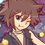# Number Theory (1st math Thailand POSN 2014)

You cannot use any theorems involving modular arithmetic.

1.) Prove the theorem that "Let $$a,b \in \mathbb{Z}$$ such that $$a \neq 0$$ or $$b \neq 0$$, we get that $$\exists x,y \in \mathbb{Z}, (a,b) = ax+by$$"

Note: $(a,b)$ is the greatest common divisor.

2.) Let $p$ be a prime number. Prove that $\exists k \in \mathbb{Z}, (p-1)! + 1 = p^{k}$ if and only if $p = 2,3,5$.

3.) Prove that if $p$ and $8p^{2}+1$ are prime numbers, then $8p^{2}+2p+1$ is also prime number.

4.) Prove that there exists infinitely many positive integer $n$ such that $10^{n}+3$ is composite.

5.) Find all such primes $p$ and positive numbers $n$ such that $n^{p} + 3^{p}$ are perfect squares.

This is the part of Thailand 1st round math POSN problems.Note by Samuraiwarm Tsunayoshi
6 years, 8 months ago

This discussion board is a place to discuss our Daily Challenges and the math and science related to those challenges. Explanations are more than just a solution — they should explain the steps and thinking strategies that you used to obtain the solution. Comments should further the discussion of math and science.

When posting on Brilliant:

• Use the emojis to react to an explanation, whether you're congratulating a job well done , or just really confused .
• Ask specific questions about the challenge or the steps in somebody's explanation. Well-posed questions can add a lot to the discussion, but posting "I don't understand!" doesn't help anyone.
• Try to contribute something new to the discussion, whether it is an extension, generalization or other idea related to the challenge.
• Stay on topic — we're all here to learn more about math and science, not to hear about your favorite get-rich-quick scheme or current world events.

MarkdownAppears as
*italics* or _italics_ italics
**bold** or __bold__ bold
- bulleted- list
• bulleted
• list
1. numbered2. list
1. numbered
2. list
Note: you must add a full line of space before and after lists for them to show up correctly
paragraph 1paragraph 2

paragraph 1

paragraph 2

[example link](https://brilliant.org)example link
> This is a quote
This is a quote
    # I indented these lines
# 4 spaces, and now they show
# up as a code block.

print "hello world"
# I indented these lines
# 4 spaces, and now they show
# up as a code block.

print "hello world"
MathAppears as
Remember to wrap math in $$ ... $$ or $ ... $ to ensure proper formatting.
2 \times 3 $2 \times 3$
2^{34} $2^{34}$
a_{i-1} $a_{i-1}$
\frac{2}{3} $\frac{2}{3}$
\sqrt{2} $\sqrt{2}$
\sum_{i=1}^3 $\sum_{i=1}^3$
\sin \theta $\sin \theta$
\boxed{123} $\boxed{123}$

## Comments

Sort by:

Top Newest

For 3, 8p^2 +1 is a multiple of 3 if p isn't equal to3 . so, p = 3 and hence forth, it becomes trivial

- 6 years, 8 months ago

Log in to reply

1.) is just the Euclidean algorithm, but I can't just freaking prove it nuuuu.

- 6 years, 8 months ago

Log in to reply

For 4, if n is of form 6k + 4 , then 10^n + 3 is a multiple of 7, hence composite

- 6 years, 8 months ago

Log in to reply

$Problem \enspace 3:$

If $p=2, \enspace 8p^2+1=8(2)^2+1=33 ;$Not prime

If $p=3, \enspace 8p^2+1=8(3)^2+1=73 \enspace$ and $\enspace 8p^2+2p+1=8(3)^2+2(3)+1=79 ;$ both are primes.

Other prime numbers can be written as $6k\pm1$.

Then, $8p^2+1 = 8(6k\pm1)^2+1=8(36k^2\pm12k+1)+1 = 3(96k^2\pm32k+3)$ ; which can't be a prime.

So only for $p=3$, $8p^2+1$ and $8p^2+2p+1$ both become prime.

- 6 years, 8 months ago

Log in to reply

×

Problem Loading...

Note Loading...

Set Loading...